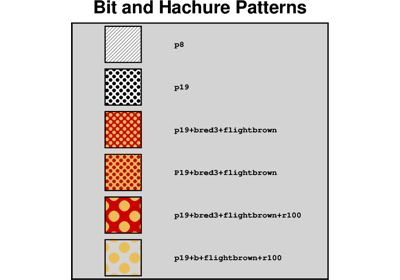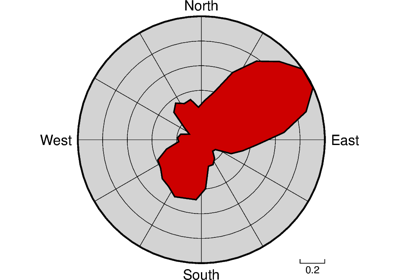# pygmt.Figure.rose

Figure.rose(data=None, length=None, azimuth=None, *, sector=None, frame=None, cmap=None, shift=None, vectors=None, no_scale=None, fill=None, inquire=None, diameter=None, labels=None, vector_params=None, alpha=None, region=None, norm=None, orientation=None, verbose=None, pen=None, scale=None, binary=None, nodata=None, find=None, header=None, incols=None, panel=None, perspective=None, transparency=None, wrap=None, **kwargs)

Plot windrose diagrams or polar histograms.

Takes a matrix, (length,azimuth) pairs, or a file name as input and plots windrose diagrams or polar histograms (sector diagram or rose diagram).

Must provide either `data` or `length` and `azimuth`.

Options include full circle and half circle plots. The outline of the windrose is drawn with the same color as MAP_DEFAULT_PEN.

Full option list at https://docs.generic-mapping-tools.org/latest/rose.html

Aliases:

• A = sector

• B = frame

• C = cmap

• D = shift

• Em = vectors

• F = no_scale

• G = fill

• I = inquire

• JX = diameter

• L = labels

• M = vector_params

• Q = alpha

• R = region

• S = norm

• T = orientation

• V = verbose

• W = pen

• Z = scale

• b = binary

• c = panel

• d = nodata

• e = find

• i = incols

• p = perspective

• t = transparency

• w = wrap

Parameters
• data (str or numpy.ndarray or pandas.DataFrame or xarray.Dataset or geopandas.GeoDataFrame) – Pass in either a file name to an ASCII data table, a 2-D `numpy.ndarray`, a `pandas.DataFrame`, an `xarray.Dataset` made up of 1-D `xarray.DataArray` data variables, or a `geopandas.GeoDataFrame` containing the tabular data. Use parameter `incols` to choose which columns are length and azimuth, respectively. If a file with only azimuths is given, use `incols` to indicate the single column with azimuths; then all lengths are set to unity (see `scale="u"` to set actual lengths to unity as well).

• length/azimuth (float or 1-D arrays) – Length and azimuth values, or arrays of length and azimuth values.

• orientation (bool) – Specify that the input data are orientation data (i.e., have a 180 degree ambiguity) instead of true 0-360 degree directions [Default is 0-360 degrees]. We compensate by counting each record twice: First as azimuth and second as azimuth +180. Ignored if `region` is given as (-90, 90) or (0, 180).

• region (str or list) – r0/r1/az0/az1 or [r0, r1, az0, az1]. Required if this is the first plot command. Specify the `region` of interest in (r, azimuth) space. Here, r0 is 0 and r1 is the maximal length in units. For az0 and az1, specify either (-90, 90) or (0, 180) for half circle plot or (0, 360) for full circle.

• diameter (str) – Set the diameter of the rose diagram. If not given, then we default to a diameter of 7.5 cm.

• sector (float or str) – Give the sector width in degrees for sector and rose diagram. Default `0` means windrose diagram. Append +r to draw rose diagram instead of sector diagram (e.g. `"10+r"`).

• norm (bool) – Normalize input radii (or bin counts if `sector` is used) by the largest value so all radii (or bin counts) range from 0 to 1.

• frame (str) – Set map boundary frame and axes attributes. Remember that x here is radial distance and y is azimuth. The ylabel may be used to plot a figure caption. The scale bar length is determined by the radial gridline spacing.

• scale (float or str) – Multiply the data radii by scale. E.g., use `scale=0.001` to convert your data from m to km. To exclude the radii from consideration, set them all to unity with `scale="u"` [Default is no scaling].

• fill (str) – Set color or pattern for filling sectors [Default is no fill].

• cmap (str) – Give a CPT. The r-value for each sector is used to look-up the sector color. Cannot be used with a rose diagram.

• pen (str) – Set pen attributes for sector outline or rose plot, e.g. `pen="0.5p"`. [Default is no outline]. To change pen used to draw vector (requires `vectors`) [Default is same as sector outline] use e.g. `pen="v0.5p"`.

• labels (str) – wlabel,elabel,slabel,nlabel. Specify labels for the 0, 90, 180, and 270 degree marks. For full-circle plot the default is `"West,East,South,North"` and for half-circle the default is `"90W,90E,-,0"`. A `"-"` in any entry disables that label (e.g. `labels="W,E,-,N"`). Use `labels=""` to disable all four labels. Note that the GMT_LANGUAGE setting will affect the words used.

• no_scale (bool) – Do NOT draw the scale length bar (`no_scale=True`). Default plots scale in lower right corner provided `frame` is used.

• shift (bool) – Shift sectors so that they are centered on the bin interval (e.g., first sector is centered on 0 degrees).

• vectors (str) – mode_file. Plot vectors showing the principal directions given in the mode_file file. Alternatively, specify `vectors` to compute and plot mean direction. See `vector_params` to control the vector attributes. Finally, to instead save the computed mean direction and other statistics, use `vectors="+wmode_file"`. The eight items saved to a single record are: mean_az, mean_r, mean_resultant, max_r, scaled_mean_r, length_sum, n, sign@alpha, where the last term is 0 or 1 depending on whether the mean resultant is significant at the level of confidence set via `alpha`.

• vector_params (str) – Used with `vectors` to modify vector parameters. For vector heads, append vector head size [Default is 0, i.e., a line]. See https://docs.generic-mapping-tools.org/latest/rose.html#vector-attributes for specifying additional attributes. If `vectors` is not given and the current plot mode is to draw a windrose diagram then using `vector_params` will add vector heads to all individual directions using the supplied attributes.

• alpha (float or str) –

Set the confidence level used to determine if the mean resultant is significant (i.e., Lord Rayleigh test for uniformity) [Default is `alpha=0.05`]. Note: The critical values are approximated [Berens, 2009] and requires at least 10 points; the critical resultants are accurate to at least 3 significant digits. For smaller data sets you should consult exact statistical tables.

Berens, P., 2009, CircStat: A MATLAB Toolbox for Circular Statistics, J. Stat. Software, 31(10), 1-21, https://doi.org/10.18637/jss.v031.i10.

• verbose (bool or str) –

Select verbosity level [Default is w], which modulates the messages written to stderr. Choose among 7 levels of verbosity:

• q - Quiet, not even fatal error messages are produced

• e - Error messages only

• w - Warnings [Default]

• t - Timings (report runtimes for time-intensive algorithms)

• i - Informational messages (same as `verbose=True`)

• c - Compatibility warnings

• d - Debugging messages

• binary (bool or str) –

i|o[ncols][type][w][+l|b]. Select native binary input (using `binary="i"`) or output (using `binary="o"`), where ncols is the number of data columns of type, which must be one of:

• c - int8_t (1-byte signed char)

• u - uint8_t (1-byte unsigned char)

• h - int16_t (2-byte signed int)

• H - uint16_t (2-byte unsigned int)

• i - int32_t (4-byte signed int)

• I - uint32_t (4-byte unsigned int)

• l - int64_t (8-byte signed int)

• L - uint64_t (8-byte unsigned int)

• f - 4-byte single-precision float

• d - 8-byte double-precision float

• x - use to skip ncols anywhere in the record

For records with mixed types, append additional comma-separated combinations of ncols type (no space). The following modifiers are supported:

• w after any item to force byte-swapping.

• +l|b to indicate that the entire data file should be read as little- or big-endian, respectively.

Full documentation is at https://docs.generic-mapping-tools.org/latest/gmt.html#bi-full.

• panel (bool or int or list) – [row,col|index]. Select a specific subplot panel. Only allowed when in subplot mode. Use `panel=True` to advance to the next panel in the selected order. Instead of row,col you may also give a scalar value index which depends on the order you set via `autolabel` when the subplot was defined. Note: row, col, and index all start at 0.

• nodata (str) – i|onodata. Substitute specific values with NaN (for tabular data). For example, `nodata="-9999"` will replace all values equal to -9999 with NaN during input and all NaN values with -9999 during output. Prepend i to the nodata value for input columns only. Prepend o to the nodata value for output columns only.

• find (str) – [~]“pattern” | [~]/regexp/[i]. Only pass records that match the given pattern or regular expressions [Default processes all records]. Prepend ~ to the pattern or regexp to instead only pass data expressions that do not match the pattern. Append i for case insensitive matching. This does not apply to headers or segment headers.

[i|o][n][+c][+d][+msegheader][+rremark][+ttitle]. Specify that input and/or output file(s) have n header records [Default is 0]. Prepend i if only the primary input should have header records. Prepend o to control the writing of header records, with the following modifiers supported:

• +d to remove existing header records.

• +c to add a header comment with column names to the output [Default is no column names].

• +r to add a remark comment to the output [Default is no comment]. The remark string may contain \n to indicate line-breaks.

• +t to add a title comment to the output [Default is no title]. The title string may contain \n to indicate line-breaks.

Blank lines and lines starting with # are always skipped.

• incols (str or 1-D array) –

Specify data columns for primary input in arbitrary order. Columns can be repeated and columns not listed will be skipped [Default reads all columns in order, starting with the first (i.e., column 0)].

• For 1-D array: specify individual columns in input order (e.g., `incols=[1,0]` for the 2nd column followed by the 1st column).

• For `str`: specify individual columns or column ranges in the format start[:inc]:stop, where inc defaults to 1 if not specified, with columns and/or column ranges separated by commas (e.g., `incols="0:2,4+l"` to input the first three columns followed by the log-transformed 5th column). To read from a given column until the end of the record, leave off stop when specifying the column range. To read trailing text, add the column t. Append the word number to t to ingest only a single word from the trailing text. Instead of specifying columns, use `incols="n"` to simply read numerical input and skip trailing text. Optionally, append one of the following modifiers to any column or column range to transform the input columns:

• +l to take the log10 of the input values.

• +d to divide the input values by the factor divisor [Default is 1].

• +s to multiple the input values by the factor scale [Default is 1].

• +o to add the given offset to the input values [Default is 0].

• perspective (list or str) – [x|y|z]azim[/elev[/zlevel]][+wlon0/lat0[/z0]][+vx0/y0]. Select perspective view and set the azimuth and elevation angle of the viewpoint [Default is `[180, 90]`]. Full documentation is at https://docs.generic-mapping-tools.org/latest/gmt.html#perspective-full.

• transparency (int or float) – Set transparency level, in [0-100] percent range [Default is `0`, i.e., opaque]. Only visible when PDF or raster format output is selected. Only the PNG format selection adds a transparency layer in the image (for further processing).

• wrap (str) –

y|a|w|d|h|m|s|cperiod[/phase][+ccol]. Convert the input x-coordinate to a cyclical coordinate, or a different column if selected via +ccol. The following cyclical coordinate transformations are supported:

• y - yearly cycle (normalized)

• a - annual cycle (monthly)

• w - weekly cycle (day)

• d - daily cycle (hour)

• h - hourly cycle (minute)

• m - minute cycle (second)

• s - second cycle (second)

• c - custom cycle (normalized)

Full documentation is at https://docs.generic-mapping-tools.org/latest/gmt.html#w-full.

## Examples using `pygmt.Figure.rose`Bit and Hachure Patterns

Bit and Hachure PatternsRose diagram

Rose diagram